In the ideal double-slit experiment, when a glass-plate (refractive index 1.5) of thickness t is introduced in the path of one of the interfering beams (wavelength λ), the intensity at the position where the central maximum occurred previously remains unchanged. The minimum thickness of the glass-plate is

(1) 2λ

(2) $\frac{2\lambda }{3}$

(3) $\frac{\lambda }{3}$

(4) λ

High Yielding Test Series + Question Bank - NEET 2020

Difficulty Level:

In the figure is shown Young’s double slit experiment. Q is the position of the first bright fringe on the right side of O. P is the 11th fringe on the other side, as measured from Q. If the wavelength of the light used is 6000 × 10–10 m, then S1B will be equal to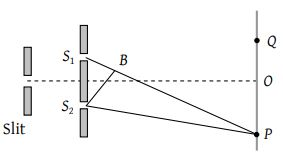(1) 6 × 10–6 m

(2) 6. 6 × 10–6 m

(3) 3.138 × 10–7 m

(4) 3.144 × 10–7 m

Concept Questions :-

Young Double slit experiment
High Yielding Test Series + Question Bank - NEET 2020

Difficulty Level:

In Young’s double slit experiment, the two slits act as coherent sources of equal amplitude A and wavelength λ. In another experiment with the same set up the two slits are of equal amplitude A and wavelength λ but are incoherent. The ratio of the intensity of light at the mid-point of the screen in the first case to that in the second case is

(1) 1 : 2

(2) 2 : 1

(3) 4 : 1

(4) 1 : 1

Concept Questions :-

Young Double slit experiment
High Yielding Test Series + Question Bank - NEET 2020

Difficulty Level:

A monochromatic beam of light falls on YDSE apparatus at some angle (say θ) as shown in figure. A thin sheet of glass is inserted in front of the lower slit S2. The central bright fringe (path difference = 0) will be obtained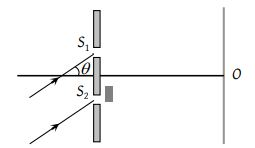(1) At O

(2) Above O

(3) Below O

(4) Anywhere depending on angle θ, thickness of plate t and refractive index of glass μ

Concept Questions :-

Young Double slit experiment
High Yielding Test Series + Question Bank - NEET 2020

Difficulty Level:

Two ideal slits S1 and S2 are at a distance d apart, and illuminated by light of wavelength λ passing through an ideal source slit S placed on the line through S2 as shown. The distance between the planes of slits and the source slit is D. A screen is held at a distance D from the plane of the slits. The minimum value of d for which there is darkness at O is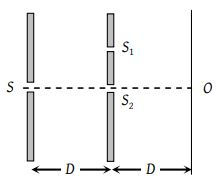(1) $\sqrt{\frac{3\lambda D}{2}}$

(2) $\sqrt{\lambda D}$

(3) $\sqrt{\frac{\lambda D}{2}}$

(4) $\sqrt{3\lambda D}$

Concept Questions :-

Young Double slit experiment
High Yielding Test Series + Question Bank - NEET 2020

Difficulty Level:

Two point sources X and Y emit waves of same frequency and speed but Y lags in phase behind X by 2πl radian. If there is a maximum in direction D the distance XO using n as an integer is given by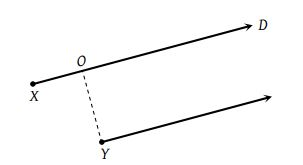(1) $\frac{\lambda }{2}\left(n-l\right)$

(2) $\lambda \left(n+l\right)$

(3) $\frac{\lambda }{2}\left(n+l\right)$

(4) $\lambda \left(n-l\right)$

Concept Questions :-

Diffraction
High Yielding Test Series + Question Bank - NEET 2020

Difficulty Level:

A beam with wavelength λ falls on a stack of partially reflecting planes with separation d. The angle θ that the beam should make with the planes so that the beams reflected from successive planes may interfere constructively is (where n =1, 2, ……)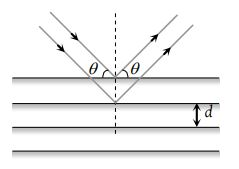(1) ${\mathrm{sin}}^{-1}\left(\frac{n\lambda }{d}\right)$

(2) ${\mathrm{tan}}^{-1}\left(\frac{n\lambda }{d}\right)$

(3) ${\mathrm{sin}}^{-1}\left(\frac{n\lambda }{2d}\right)$

(4) ${\mathrm{cos}}^{-1}\left(\frac{n\lambda }{2d}\right)$

Concept Questions :-

Interference vs Diffraction
High Yielding Test Series + Question Bank - NEET 2020

Difficulty Level:

Two coherent sources separated by distance d are radiating in phase having wavelength λ. A detector moves in a big circle around the two sources in the plane of the two sources. The angular position of n = 4 interference maxima is given as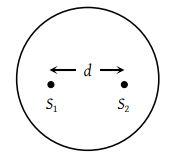(1) ${\mathrm{sin}}^{-1}\frac{n\lambda }{d}$

(2) ${\mathrm{cos}}^{-1}\frac{4\lambda }{d}$

(3) ${\mathrm{tan}}^{-1}\frac{d}{4\lambda }$

(4) ${\mathrm{cos}}^{-1}\frac{\lambda }{4d}$

Concept Questions :-

Interference vs Diffraction
High Yielding Test Series + Question Bank - NEET 2020

Difficulty Level:

A circular disc is placed in front of a narrow source. When the point of observation is 2 m from the disc, then it covers first HPZ. The intensity at this point is I. When the point of observation is 25 cm from the disc then intensity will be

(1) ${\left(\frac{{R}_{6}}{{R}_{2}}\right)}^{2}I$

(2) ${\left(\frac{{R}_{7}}{{R}_{2}}\right)}^{2}I$

(3) ${\left(\frac{{R}_{8}}{{R}_{2}}\right)}^{2}I$

(4) ${\left(\frac{{R}_{9}}{{R}_{2}}\right)}^{2}I$

Concept Questions :-

Interference vs Diffraction
High Yielding Test Series + Question Bank - NEET 2020

Difficulty Level:

In a single slit diffraction of light of wavelength λ by a slit of width e, the size of the central maximum on a screen at a distance b is

(1) $2b\lambda +e$

(2) $\frac{2b\lambda }{e}$

(3) $\frac{2b\lambda }{e}+e$

(4) $\frac{2b\lambda }{e}-e$

Concept Questions :-

Diffraction